Formal charge formula homework Help at TutorEye

# Best Homework Help For Formal charge formula

Our experts are available 24/7 to help you with Formal charge formula homework problems!

## Top Questions

ation. Here is the review questions that are similar to the ones I will have. Thanks https://dal.brightspace.com/d2l/common/assets/pdfjs/1.0.0.30/web/viewer.html?file=%2Fcontent%2Fenforced%2F187164-10382.202210%2FUnit%2520Exam%25203%2520Review%2520Questions%2520-%2520Solutions.pdf%3Fd2lSessionVal%3DaLMtlo7qT0DZ0jhwkJtUqnXJL%26ou%3D187164&lang=en-us&container=d2l-fileviewer-rendered-pdf&fullscreen=d2l-fileviewer-rendered-pdf-dialog&height=913#page=1
View More

## Formal charge formula

The formal charge can be calculated using following formula-
Formal charge = (No. of valence electrons on an atom) - (Non-bonded electrons on atom + number of bonds)

## Formal charge formula Sample Questions

Question 1: What is the most stable formal charge-

a) +1                          b) -1
c) 0                            d) 3

Answer: Option c) 0

Explanation: An atom tries to complete its octet, and when it has a preferred number of bonds to complete the octet, its formal charge is zero (0). When the number of electrons and protons in an atom is equal, the formal charge on an atom is 0. This is the most stable state of the atom.

Question 2: Carbon can have formal charge of-

a) 0                        b) +1
c) -1                       d) All of the above.

Answer: Option d) All of the above.

Explanation: Carbon is a tetravalent atom and forms four covalent bonds. In all the molecules where carbon has four bonds, its formal charge is zero.There are few exceptions, when a carbon has only three bonds and no lone pairs of electrons it acquires a formal charge of +1 and is called carbocation. When the carbon atom has three bonds and a lone pair of electrons it acquires -1 formal charge and is called carbanions.

Question 3: In the lewis structure of ClF the formal charge on Cl and F is respectively-

a) 0,0                           b) 0,1
c) 1,0                           d) 1,1

Answer: Option a) 0,0

Explanation: Both F and Cl have 7 valence electrons, one is used in bonding with each other while rest 6 are non-bonded.
Formal charge = (No. of valence electrons on an atom) - (Non-bonded electrons on atom + number of bonds)
Formal charge = (7)-(6+1)=0

Question 4: The number of valence electrons in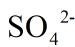are-

a) 26                         b) 28
c) 30                         d) 32

Answer: Option d) 32

Explanation: There is one sulphur atom and four oxygen atoms in a sulphate ion. There are six valence electrons in each sulphur and each oxygen atom, as there are total of four oxygen atoms the total number of valence electrons for a sulphur and four oxygen atoms are. The -2 charge onrepresents two extra electrons making the total count of valence electrons 30+2=32.

Question 5: What is the charge on N in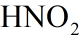?

a) 0                        b) +1
c) +2                      d) +3

Answer: Option a) 0

Explanation: Formal charge = (No. of valence electrons on an atom) - (Non-bonded electrons on atom + number of bonds)
There are 5 valence electrons in the N atom. Three of these valence electrons are used in single bonding with one of the O atoms and double bonding with the other one. The remaining two electrons are non bonded.
Formal charge = 5-2+3= 0

Question 6: What is the formal charge on fluorine atoms in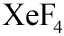molecule.

a) +1                          b) 0
c) -1                           d) +2

Answer: Option b) 0

Explanation: Formal charge = (No. of valence electrons on an atom) - (Non-bonded electrons on atom + number of bonds)
F atom has 7 valence electrons, in aEach F atom forms a single bond with Xe and the rest 6 electrons are non-bonded.
Formal charge =7-(6+1)=0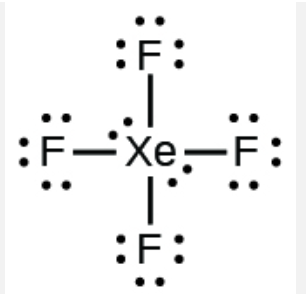Question 7: What is the formal charge on O atom in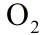.

a) -1                        b) +1
c) +2                       d) 0

Answer: Option d)  0

Explanation: O has 6 valence electrons and it uses two of them in double bonding with each other and 4 electrons are present as lone pairs on each oxygen atom.
Formal charge = (No. of valence electrons on an atom) - (Non-bonded electrons on atom + number of bonds)
Formal charge= 6-(4+2)=0

Question 8: Formal charge of S atom in bisulphate ion is-

a) +1                          b) +2
c) +4                          d) +6

Answer: Option b) +2

Explanation: Formal charge = (No. of valence electrons on an atom) - (Non-bonded electrons on atom + number of bonds)
S atom has 6 valence electrons and forms six bonds in bisulphate ion-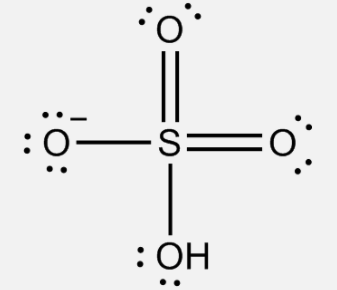Formal charge= 6-(0+6)=0

Question 9: What is the formal charge formula?

a) (Valence electrons + bond pairs) - Lone pairs                                                  b) Lone pairs + (Non-bonded electrons - bond pairs)
c) Valence electrons - Non-bonded electrons + number of bonds                        d) (Bond pairs-lone pairs) + Valence electrons

Answer: Option c) Valence electrons - Non-bonded electrons + number of bonds

Explanation: The formal charge can be calculated using formula-
Formal charge = (No. of valence electrons on an atom) - (Non-bonded electrons on atom + number of bonds)

Question 10: What are valence electrons-

a) Total electrons in an atom                           b) Electrons in outermost shell
c) Noble gases                                                d) Electrons in outermost subshell

Answer: Option b) Electrons in outermost shell

Explanation: Valence electrons are electrons present in the outermost shell of an atom, and can participate in the formation of a chemical bond with other atoms.

## Frequently Asked Questions

### How to find the formal charge of a Lewis formula?

The formal charge can be calculated using formula-

Formal charge = (No. of valence electrons on an atom) - (Non-bonded electrons on atom + number of bonds)

For example- For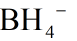No. of valence electrons for boron is 3.
The No. of non-bonded electrons is zero.
The No. of bonds around boron is 4.
So formal charge = 3 – (0 + 4)  = 3 – 4  = –1

### What is the formal charge formula?

Formal charge can be calculated using following formula
Formal charge = (No. of valence electrons on an atom) - (Non-bonded electrons on atom + number of bonds)

### What is the best formal charge?

An atom tends to complete its octet, and when it has a preferred number of bonds to achieve the octet, its formal charge is zero (0). The formal charge on an atom is 0 when the number of electrons and protons in an atom is equal. This is the most stable state of the atom.

### Can carbon have a formal charge?

Carbon is a tetravalent atom and forms four covalent bonds. In all the molecules where carbon has four bonds, its formal charge is zero.There are few exceptions, when a carbon has only three bonds and no lone pairs of electrons it acquires a formal charge of +1 and is called carbocation. When the carbon atom has three bonds and a lone pair of electrons it acquires -1 formal charge and is called carbanions.